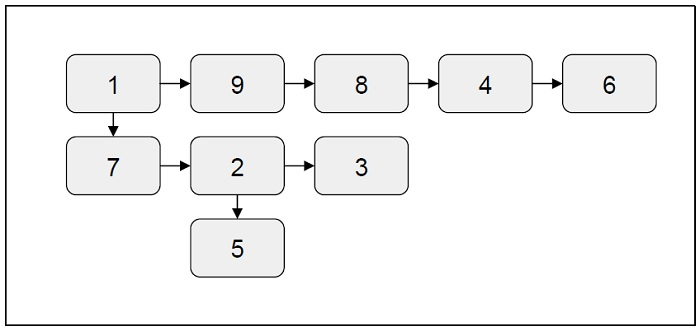# Flatten a multilevel linked list in C++

In this problem, we are given a multilevel linked list. Our task is to create a program to flatten a multilevel linked list.

The flattening operation is done in such a way that the first level nodes will occur first in the linked list and then the second level nodes will occur.

Multilevel linked list is a multi-dimensional data structure in which every node of the linked list has two link pointers, one a link to the next node and one to the child list with one or more nodes. This child pointer may or may not point to other list nodes.

## ExampleLet’s take an example to understand the problem

InputOutput

1-> 9-> 8 -> 4 -> 6-> 7-> 2-> 3-> 5

## Solution Approach

A simple solution to the problem is by using doing a level order traversal type of algorithm. We will be traversing the linked list starting from the first node and traversing all the nodes at the same level. If any child pointer is present for a node, move it to the end of the current linked list using the tail pointer. Then recursively perform the same traversal for each child node of the linked list. The below program will elaborate the logic better.

## Example

Program to illustrate the working of our solution

#include <bits/stdc++.h>
using namespace std;

#define SIZE(arr) (sizeof(arr)/sizeof(arr))

class Node{
public:
int data;
Node *next;
Node *child;
};
Node *createList(int *arr, int n){
Node *p;
int i;
for (i = 0; i < n; ++i){
head = p = new Node();
else{
p->next = new Node();
p = p->next;
}
p->data = arr[i];
p->next = p->child = NULL;
}
}
Node *createList(void){
int arr1[] = {1, 9, 8, 4, 6};
int arr2[] = {7, 3, 2};
int arr3[] = {5};
}
return;
Node *tmp;
while (tail->next != NULL)
tail = tail->next;
while (cur != tail){
if (cur->child){
tail->next = cur->child;
tmp = cur->child;
while (tmp->next)
tmp = tmp->next;
tail = tmp;
}
cur = cur->next;
}
}
int main(void){
}
The flattened Linked List is 1 9 8 4 6 7 3 2 5